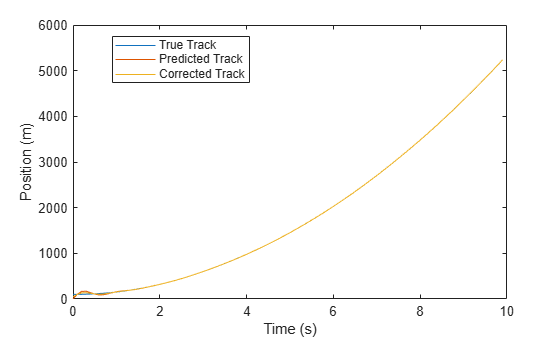# AlphaBetaFilter

Alpha-beta filter for object tracking

## Description

The `AlphaBetaFilter` object represents an alpha-beta filter designed for object tracking. Use this tracker for platforms that follow a linear motion model and have a linear measurement model. Linear motion is defined by constant velocity or constant acceleration. Use the filter to predict the future location of an object, to reduce noise for a detected location, or to help associate multiple objects with their tracks.

## Creation

### Syntax

``abf = AlphaBetaFilter``
``abf = AlphaBetaFilter(Name,Value,...)``

### Description

````abf = AlphaBetaFilter` creates an alpha-beta filter for a discrete time, 2-D constant velocity system. The motion model of the filter corresponds to setting the `MotionModel` property to `'2D Constant Velocity'`. In this case, the filter state takes the form `[x; vx; y; vy]`.```

example

````abf = AlphaBetaFilter(Name,Value,...)` specifies the properties of the filter using one or more `Name,Value` pair arguments. Any unspecified properties take default values.```

## Properties

expand all

Model of target motion, specified as a character vector or string. Specifying `1D`, `2D` or `3D` sets the dimensions of the targets motion. Specifying `Constant Velocity` assumes that the target motion has constant velocity at each simulation step. Specifying `Constant Acceleration` assumes that the target motion has constant acceleration at each simulation step.

Data Types: `char` | `string`

Filter state, specified as a scalar or a real-valued M-element vector. A scalar input is extended to an M-element vector. The state vector is the concatenated states from each dimension.

The state vectors for each motion model are column vectors:

 MotionModel Property State Vector `'1D Constant Velocity'` `[x; vx]` `'2D Constant Velocity'` `[x; vx; y; vy]` `'3D Constant Velocity'` `[x; vx; y; vy; z; vz]` `'1D Constant Acceleration'` `[x; vx; ax]` `'2D Constant Acceleration'` `[x; vx; ax; y; vy; ay]` `'3D Constant Acceleration'` `[x; vx; ax; y; vy; ay; z; vz; az]`

where, for example, `vx` denotes velocity in the x-direction and `ax` denotes acceleration in the x-direction.

Example: `[200;0.2;150;0.1;0;0.25]`

Data Types: `double`

State error covariance, specified as an M-by-M matrix where M is the size of the filter state. A scalar input is extended to an M-by-M matrix. The covariance matrix represents the uncertainty in the filter state.

Example: `eye(6)`

Process noise covariance, specified as a scalar or an D-by-D matrix where D is the dimensionality of motion. For example, if `MotionModel` is `'2D Constant Velocity`, then D = 2. A scalar input is extended to an D-by-D matrix.

Example: `[20 0.1; 0.1 1]`

Measurement noise covariance, specified as a scalar or an D-by-D matrix where D is the dimensionality of motion. For example, if `MotionModel` is `'2D Constant Velocity`, then D = 2. A scalar input is extended to an M-by-M matrix.

Example: `[20 0.1; 0.1 1]`

Alpha-beta filter coefficients, specified as a scalar or row vector of real values. Any scalar input is extended to a row vector. If you specify constant velocity in the `MotionModel` property, the coefficients are ```[alpha beta]```. If you specify constant acceleration in the `MotionModel` property, the coefficients are ```[alpha beta gamma]```.

Example: `[20 0.1]`

## Object Functions

 `predict` Predict the state and state estimation error covariance `correct` Correct the state and state estimation error covariance `distance` Distances between measurements and predicted measurements `likelihood` Likelihood of measurement `clone` Create identical object

## Examples

collapse all

Apply the alpha-beta filter to track a target moving at constant velocity along the x-axis.

```T = 0.1; V0 = 100; N = 100; plat = phased.Platform('MotionModel','Velocity', ... 'VelocitySource','Input port','InitialPosition',[100;0;0]); abfilt = phased.AlphaBetaFilter('MotionModel','1D Constant Velocity'); Z = zeros(1,N); Zp = zeros(1,N); Zc = zeros(1,N); for m = 1:N pos = plat(T,[100+20*randn;0;0]); Z(m) = pos(1); [~,~,Zp(m)] = predict(abfilt,T); [~,~,Zc(m)] = correct(abfilt,Z(m)); end t = (0:N-1)*T; plot(t,Z,t,Zp,t,Zc) xlabel('Time (s)') ylabel('Position (m)') legend('True Track','Predicted Track','Corrected Track', ... 'Location','Best')```Apply the alpha-beta filter to track a target moving at constant acceleration along the x-axis.

```T = 0.1; a0 = 100; N = 100; plat = phased.Platform('MotionModel','Acceleration', ... 'AccelerationSource','Input port','InitialPosition',[100;0;0]); abfilt = phased.AlphaBetaFilter( ... 'MotionModel','1D Constant Acceleration', ... 'Coefficients',[0.5 0.5 0.1]); Z = zeros(1,N); Zp = zeros(1,N); Zc = zeros(1,N); for m = 1:N pos = plat(T,[100+20*randn;0;0]); Z(m) = pos(1); [~,~,Zp(m)] = predict(abfilt,T); [~,~,Zc(m)] = correct(abfilt,Z(m)); end t = (0:N-1)*T; plot(t,Z,t,Zp,t,Zc) xlabel('Time (s)') ylabel('Position (m)'); legend('True Track','Predicted Track','Corrected Track', ... 'Location','Best');```Apply the alpha-beta filter to track a target moving at constant velocity in three dimensions.

```T = 0.1; V0 = 100; N = 100; plat = phased.Platform('MotionModel','Velocity', ... 'VelocitySource','Input port','InitialPosition',[100;0;0]); abfilt = phased.AlphaBetaFilter('MotionModel', ... '3D Constant Velocity','State',zeros(6,1)); Z = zeros(3,N); Zp = zeros(3,N); Zc = zeros(3,N); for m = 1:N Z(:,m) = plat(T,[V0+20*randn;0;0]); [~,~,Zp(:,m)] = predict(abfilt,T); [~,~,Zc(:,m)] = correct(abfilt,Z(:,m)); end t = (0:N-1)*T; plot(t,Z(1,:),t,Zp(1,:),t,Zc(1,:)) xlabel('Time (s)') ylabel('Position along X (m)') legend('True Track','Predicted Track','Corrected Track', ... 'Location','Best')```## Extended Capabilities

### C/C++ Code GenerationGenerate C and C++ code using MATLAB® Coder™.

Introduced in R2018b